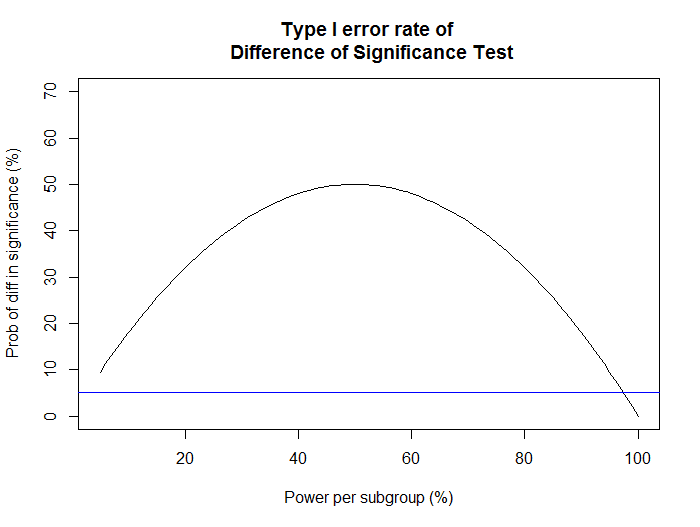## Wednesday, January 13, 2016

### "Differences of Significance" fallacy is popular because it increases Type I error

We all recognize that a difference of significance is not the same as a significant difference. That is, if men show a statistically significant (p < .05) response to some manipulation while women do not (p > .05), that does not imply that there is evidence for a difference in how men and women respond to the manipulation.

The test that should be used, of course, is the Significant Difference Test. One estimates the interaction term and its standard error, then checks the p-value representing how unusual it would be if the true value were zero. If p < .05, one concludes the two subgroups have different responses.

The incorrect test is the Differences of Significance Test. In that test, one checks the p-values for the manipulation in each subgroup and concludes a difference between subgroups if one has p < .05 and the other has p > .05.

We've seen the scientific community taking a firmer stance on people mistaking the Difference of Significance for the Significant Difference. Last year we saw Psych Science retract a paper because its core argument relied upon a Difference of Significance Test.

Why do people make this mistake? Why do we still make it, 10 years after Gelman and Stern?

My suspicion is that the Differences of Significance Test gets (unknowingly) used because it suffers from much higher Type I error rates, which allows for greater publication rates and more nuanced story-telling than is appropriate.

Let's think about two subgroups of equal size. We purport to be testing for an interaction: the two subgroups are expected to have different responses to the manipulation. We should be reporting the manipulation × subgroup interaction which, when done properly, has the nominal Type I error rate of 5%. Instead, we will look to see if one group has a significant effect of manipulation while the other is not. If so, we'll call it a success.

Assuming the two subgroups have equal size and there is no interaction, each subgroup has the same chance of having a statistically significant effect of manipulation. So the probability of getting one significant effect and one nonsignificant effect is simply the probability of getting one success on two Bernoulli trials with (Power)% success rate.5% Type 1 error rate of correct test shown as blue line.

As you can see, the Type I error rate of this improper test is very high, peaking at a whopping 50% when each subgroup has 50% power.  And this doesn't even require any questionable research practices like optional stopping or flexible outlier treatment!

Of course, one can obtain Type I error rates for this (again, improper) test by running unequal subgroups for unequal power. If group 1 is large and has 80% power to detect the effect, while group 2 is small and has only 20% power to detect, then one will find a difference in significance 68% of the time.

Obviously everybody knows the Difference of Significance Test is wrong and bad and they should be using and looking for the Significant Difference Test. But I wanted to illustrate just how bad the problem can actually be. As you can see, this isn't just nitpicking -- it can be the cause of a tenfold increase in Type I error rates.

You might be tempted to call that.... a significant difference. (hyuk hyuk hyuk)

1.2.1.